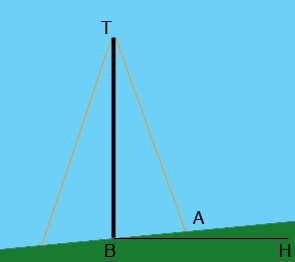SEARCH HOMEMath Central Quandaries & QueriesQuestion from larissa, a student: a radio tower 500 feet high is located on the side of a hill ( the hill has an inclination to the horizontal of 5 degrees.) How long should two guy wires be if they are connected to the top of the tower and are secured at two points 100 feet directly above ( up the hill ) and directly below the base of the tower?Hi Larissa,

I assumed that the tower is vertical.The line BH is horizontal so the measure of the angle ABH is 5o. Hence the measure of the angle TBA is 85o. You know the lengths of TB and BA so you can use the Law of Cosines to find the length of AT.

I hope this helps,
HarleyMath Central is supported by the University of Regina and The Pacific Institute for the Mathematical Sciences.